# 使用C#版OpenCV进行圆心求取

OpenCVSharp是OpenCV的.NET wrapper，是一名日本工程师开发的，项目地址为：https://github.com/shimat/opencvsharp。

## 1.OpenCVSharp的下载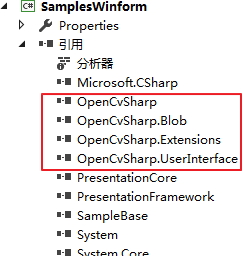## 2.拟合圆并求取圆心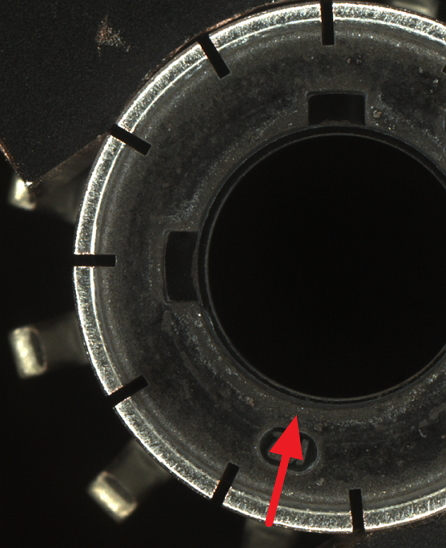using OpenCvSharp;

namespace FitCircleDemo
{
public class CircleFit
{
public  Mat Run(string imgPath)
{
//读取图片
//显示图片
//Cv2.ImShow("Input Image", img);

//转换成灰度图
Mat gray = img.CvtColor(ColorConversionCodes.BGR2GRAY);

//阈值操作 阈值参数可以用一些可视化工具来调试得到
Mat ThresholdImg = gray.Threshold(11, 255,  ThresholdTypes.Binary);
Cv2.ImShow("Threshold", ThresholdImg);

//降噪
//方法一：高斯变化
//Mat gaussImg= ThresholdImg.GaussianBlur(new Size(5, 5), 0.8);
//Cv2.ImShow("GaussianBlur", gaussImg);
//方法二：中值滤波降噪
//Mat medianImg = ThresholdImg.MedianBlur(5);
//Cv2.ImShow("MedianBlur", medianImg);

//方法三：膨胀+腐蚀
////膨胀处理
//Mat kernel = new Mat(15, 15, MatType.CV_8UC1);
//Mat DilateImg = ThresholdImg.Dilate(kernel);
////腐蚀处理
//Mat binary = DilateImg.Erode(kernel);
////显示中间结果
//Cv2.ImShow("Dilate & Erode", binary);
Mat element = Cv2.GetStructuringElement(MorphShapes.Ellipse, new Size(3, 3));
Mat openImg = ThresholdImg.MorphologyEx(MorphTypes.Open, element);
Cv2.ImShow("Dilate & Erode", openImg);

//设置感兴趣的区域
int x = 150, y = 100, w = 294, h = 337;
Rect roi = new Rect(x, y, w, h);
Mat ROIimg = new Mat(openImg, roi);
//Cv2.ImShow("ROI Image", ROIimg);

//寻找图像轮廓
Point[][] contours;
HierarchyIndex[] hierachy;
Cv2.FindContours(ROIimg, out contours, out hierachy, RetrievalModes.List, ContourApproximationModes.ApproxTC89KCOS);
//根据找到的轮廓点，拟合椭圆
for (int i = 0; i < contours.Length; i++)
{
//拟合函数必须至少5个点，少于则不拟合
if (contours[i].Length < 5) continue;
//椭圆拟合
var rrt = Cv2.FitEllipse(contours[i]);

//ROI复原
rrt.Center.X += x;
rrt.Center.Y += y;

//画椭圆
Cv2.Ellipse(img, rrt, new Scalar(0, 0, 255), 2, LineTypes.AntiAlias);
//画圆心
Cv2.Circle(img, (int)(rrt.Center.X), (int)(rrt.Center.Y), 4, new Scalar(255, 0, 0), -1, LineTypes.Link8, 0);
}

Cv2.ImShow("Fit Circle", img);
return img;
}
}
}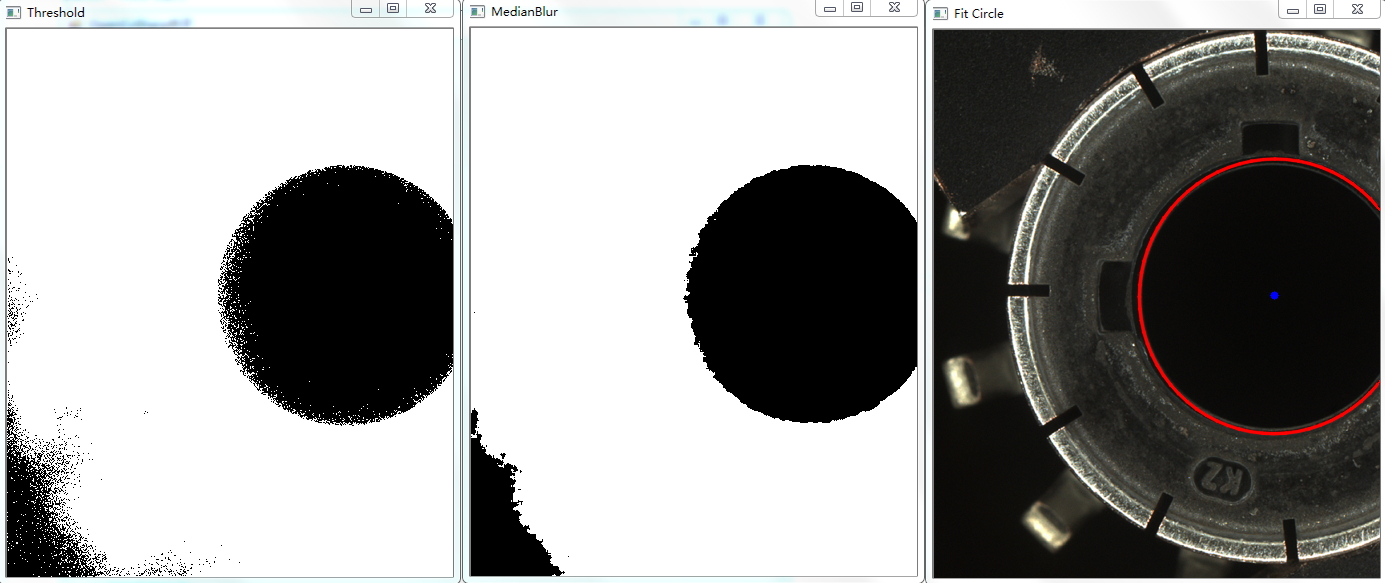1.腐蚀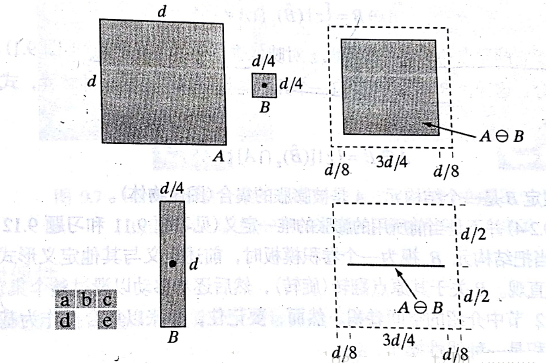2.膨胀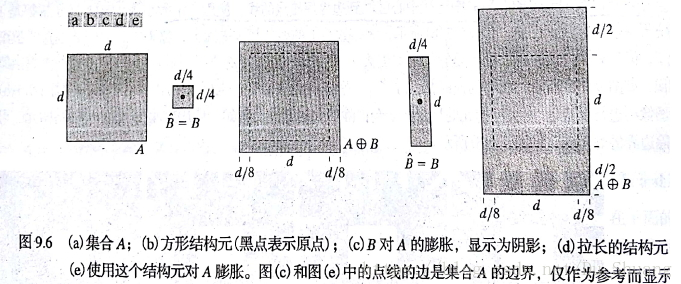B的锚点在A的边界进行扫描，A与B的并集就是B对A进行膨胀的结果。

posted @ 2019-05-06 14:50  小y  阅读(2537)  评论(0编辑  收藏